# NCERT Solutions for Class 10 Math Chapter 3 Pair of Linear Equation in Two Variables

NCERT Solution for Class 10 Maths Chapter 3

Students will learn how to solve problems involving the concept in Chapter 3 Pair of Linear Equations in Two Variables. Mathematics is a subject which is most demands of practice. The children who give the board exam examinations in 10th grade can use the NCERT Solutions Class 10 as a study guide. The Chapter Pair of Linear Equations in Two Variables solutions include step-by-step solutions to all of the NCERT textbook's math questions by our expert teachers.

A linear equation with two variables x and y is an equation of the form ax + by + c = 0, where a, b, and c are real values and a and b are not both zero.

The NCERT Solutions for Class 10 Math Chapter 3 further explains that a solution to such an equation is a pair of values, one for x and y, that equalize the two sides of the equation. Students also learn that each equation answer is represented by a point on a line.

NCERT Solutions for Class 10 Math Chapter 3 – Pair of Linear Equations in Two Variables where students get to learn multiple theories and its solutions.

This chapter covers a wide range of issues concerning linear equations in two variables. There are so many topics covered in this chapter:

3.1 The Beginning

You have already taught in your school about Linear Equations in Two Variables in previous classes. You have learned that a two-variable linear equation has an many numbers of solutions.  You can easily understand the Linear Equations in Two Variables will be reviewed and expanded in this chapter to include Pairs of Linear Equations in Two Variables.

3.2 Pair of linear equations in Two-variable

A linear equation with two variables x and y is defined as an equation with the form ax + by + c = 0, where a, b, and c are real numbers and a and b are not zeros. A pair of values, one for x and the other for y, that make the two sides of the equation are equal is the solution to such a problem. In this chapter we covered large number of geometrical representations of a pair of linear equations in two variables is also discussed, along with appropriate examples which can easily understood.

3.3 The Solution of a Pair of Linear Equations by Graphical Method

You can also saw how a pair of linear equations can be graphically represented by two lines in the previous section.

You have noticed that the lines can overlap, parallelize, or coincide. From a geometrical standpoint, you will learn how to solve it in each scenario in this part.

3.4 Solving a Pair of Linear Equations Using Algebraic Method

We looked at how to solve a pair of linear equations graphically in the previous section. The graphical method is even hard in several situations. Various algebraic approaches, such as the Substitution Method, Elimination Method, and Cross – Multiplication Method, will be discussed in this topic. For a better understanding, each subtopic is thoroughly discussed with relevant instances.

3.5 Equations in Two Variables Reduced to a Pair of Linear Equations

In this section, we'll look at how to solve such pairs. a set of nonlinear equations that can be converted to linear form by making certain appropriate modifications Some examples relevant to the subtopic are used to explain the procedure.

3.6 Conclusion

The Summary section contains the most important aspects to remember while answering the chapter Pair of Linear Equations in Two Variables exercise questions. All these points in this section will assist you in reviewing all of the concepts covered in the chapter.

A pair of linear equations in two variables is a set of two linear equations with same two variables. Graphically and algebraically, the pair of linear equations in two variables can be represented. These two lines can be used to depict the graph:

• The pair of equations is said to be consistent if the lines intersect at a point.
• The pair of equations is dependent if the lines coincide.
• If a pair of equations is inconsistent then the lines are parallel.

To solve the question of pair of linear equations in two variables algebraically, we can apply the following methods:

• Method of substitution
• Method of elimination
• Method of cross-multiplication

Why NCERT Solutions for Class 10 Math is important to go with?

Chapter 3 - Pair of Linear Equations in Two Variables have the following features.

• Subject specialists develop NCERT Solutions.
• The responses are true and come after a lot of brainstorming.
• There are questions on all of the major themes in this section.

The solutions to the NCERT Class 10 Math Chapter 3- Pair of Linear Equations in Two Variables comprises of, where our faculties have organized the NCERT Class 10 Math Solutions in a clear manner to help students to enhance their problem-solving skills. Students can use study materials to get a better understanding of Pair of Linear Equations in Two Variables.

NCERT Solution for Class 10 Maths Chapter 3: Frequently Asked Questions

1. What are the most significant themes in NCERT Solutions for Class 10 Math Chapter 3 in terms of exam preparation?

The substitution method, elimination method, and cross-multiplication method of a pair of linear equations in two variables are all covered in NCERT Solutions for Class 10 Math Chapter 3.

By resolving these students can do well in their Class 10 board exams by completing problems based on each topic with example and their solution.

1. Is it required to understand all three strategies in NCERT Solutions for Class 10 Math Chapter 3 to solve pairs of linear equations in two variables?

Yes, all three methods for solving a set of linear equations in two variables in NCERT Solutions for Class 10 Math Chapter 3 are required. This will be continued in higher education, and it is possible that they will appear in their Class 10 finals. These ideas are described in detail in by Oswaal CBSE Class 10 books. As a result, the main goal of these Class 10 books are experts-formulated answers is to impart knowledge on the fundamental parts of math, which helps students understand each idea properly.

1. How can I begin reading Chapter 3 of NCERT Solutions for Class 10 Math?

To begin, use CBSE Question Bank Class 10 to learn and comprehend the definition of linear equations. Then, at our website, look over the answers supplied by their professionals. By completing these activities, students will gain a complete understanding of all of the ideas covered in Chapter 3 of the NCERT Solutions for Class 10 Math.

 Extra Information: NCERT Exemplar for Class 10 Maths Chapter 3 CBSE Notes for Class 10 Maths Chapter 3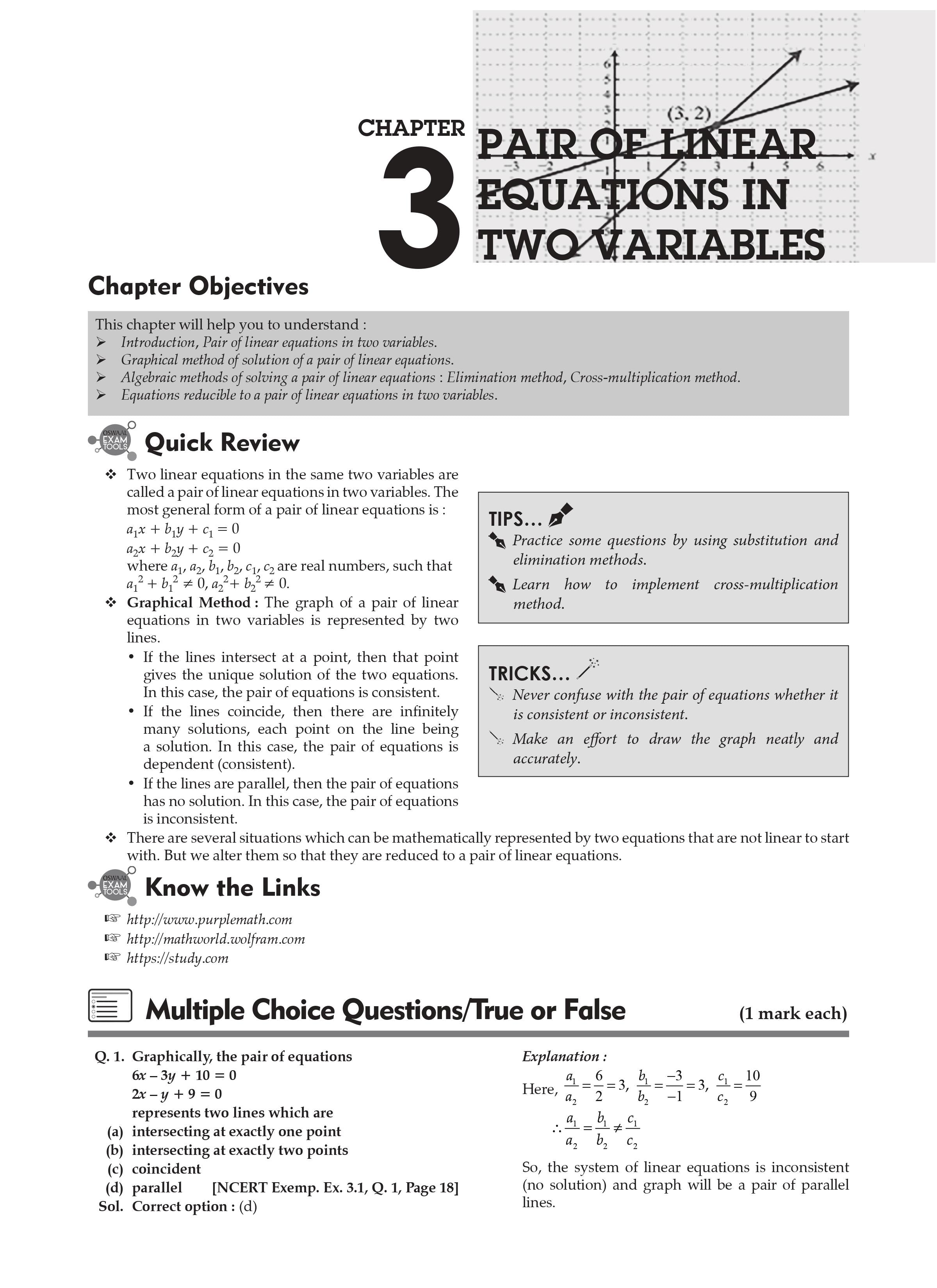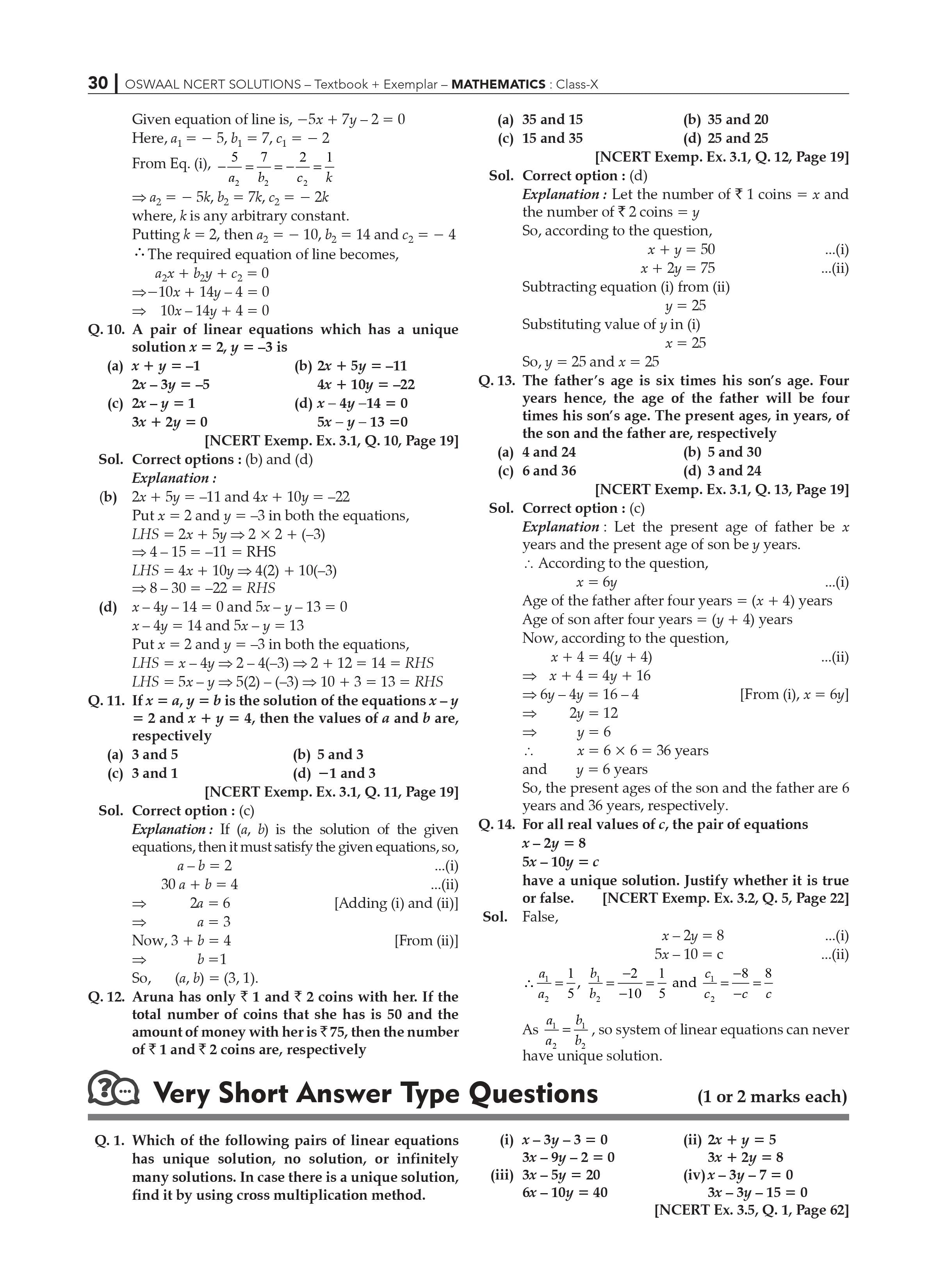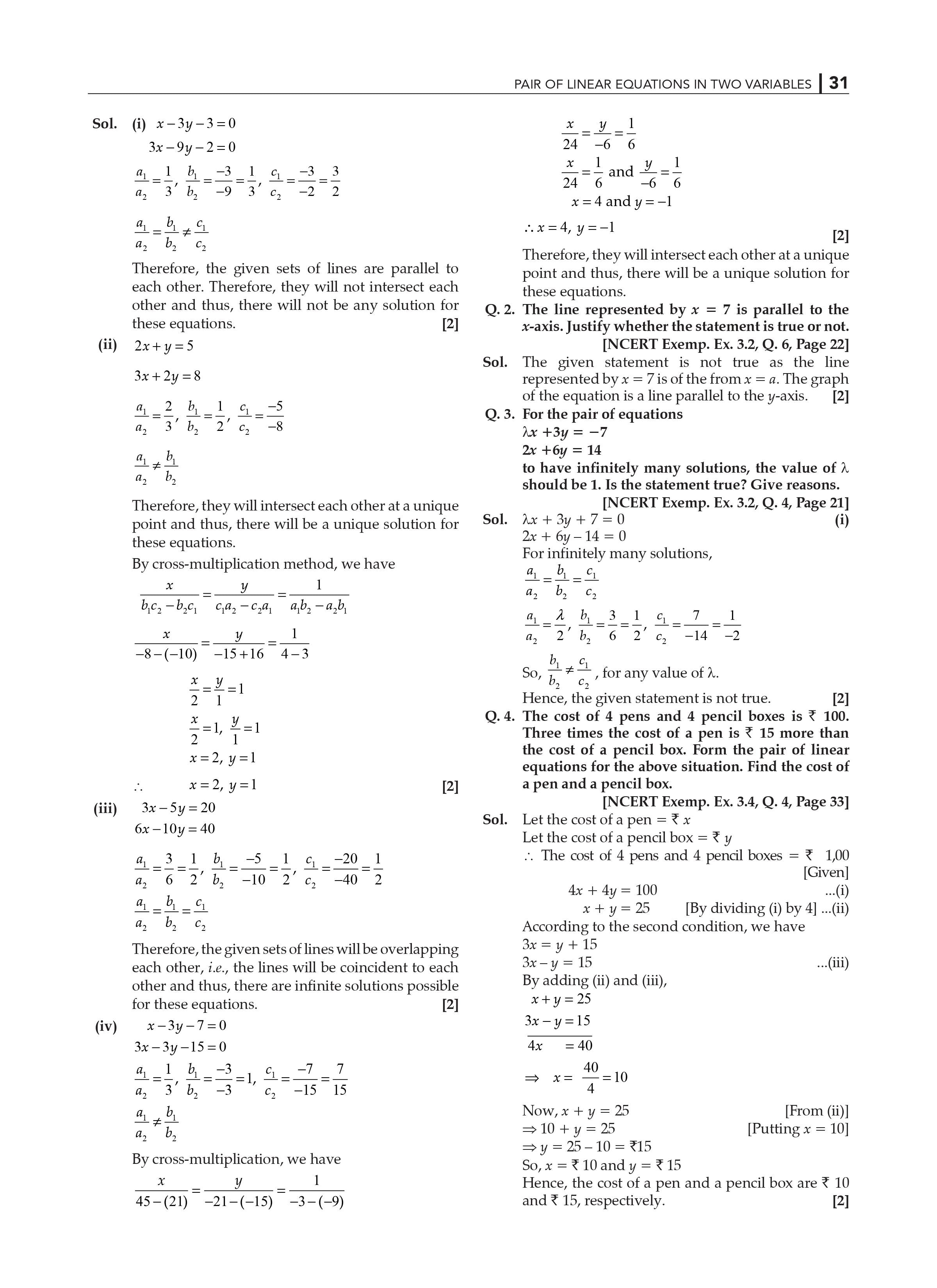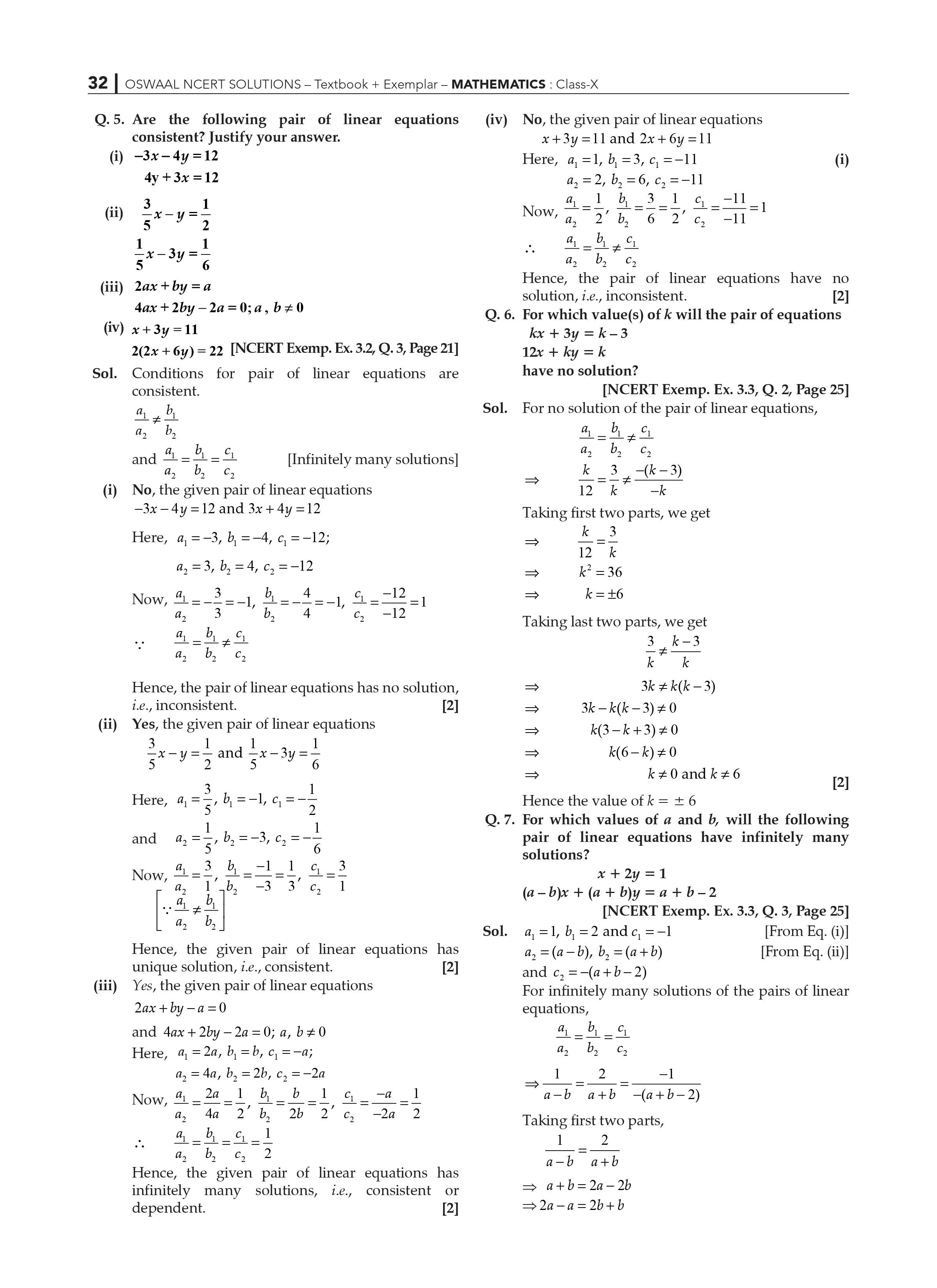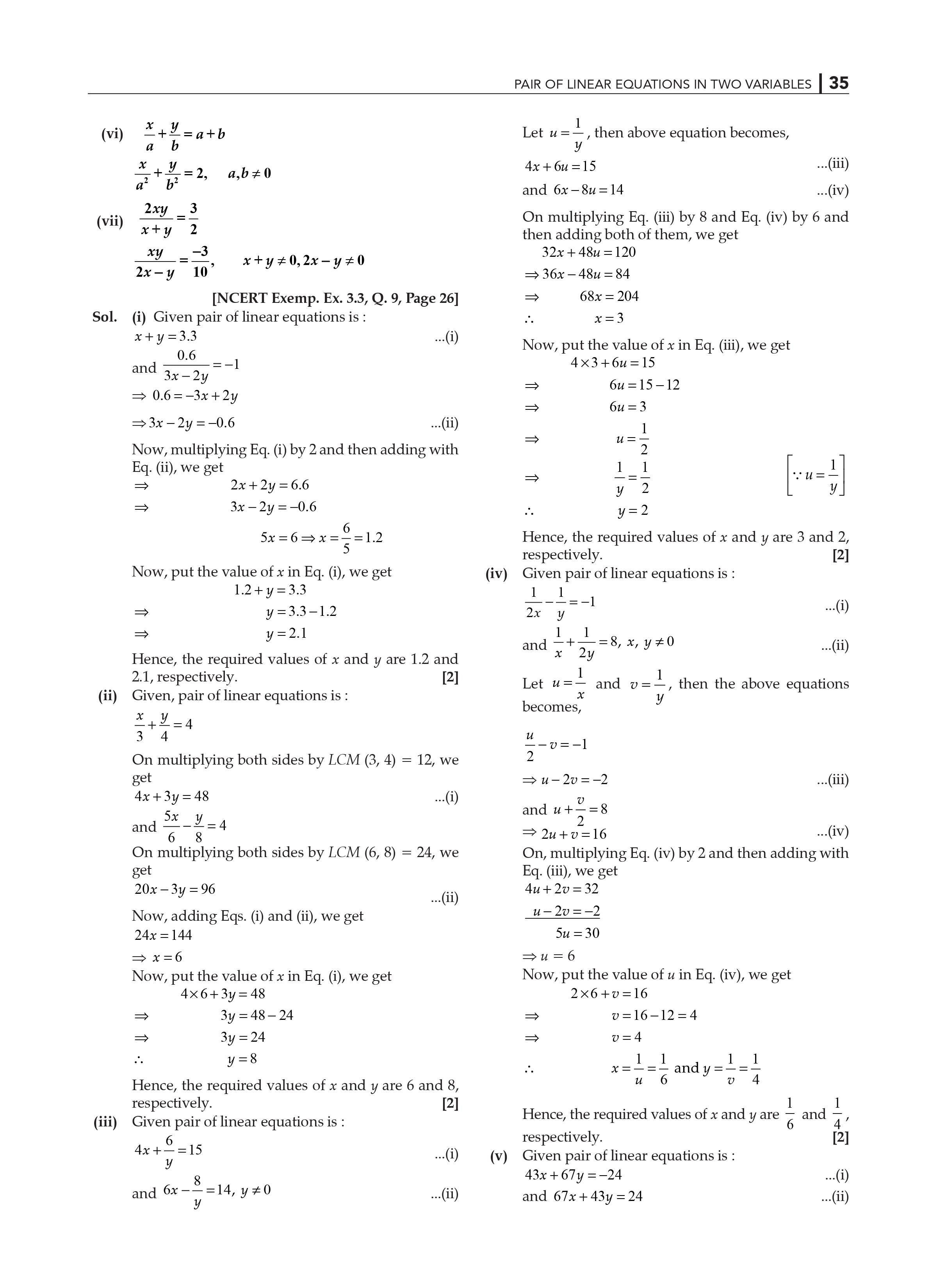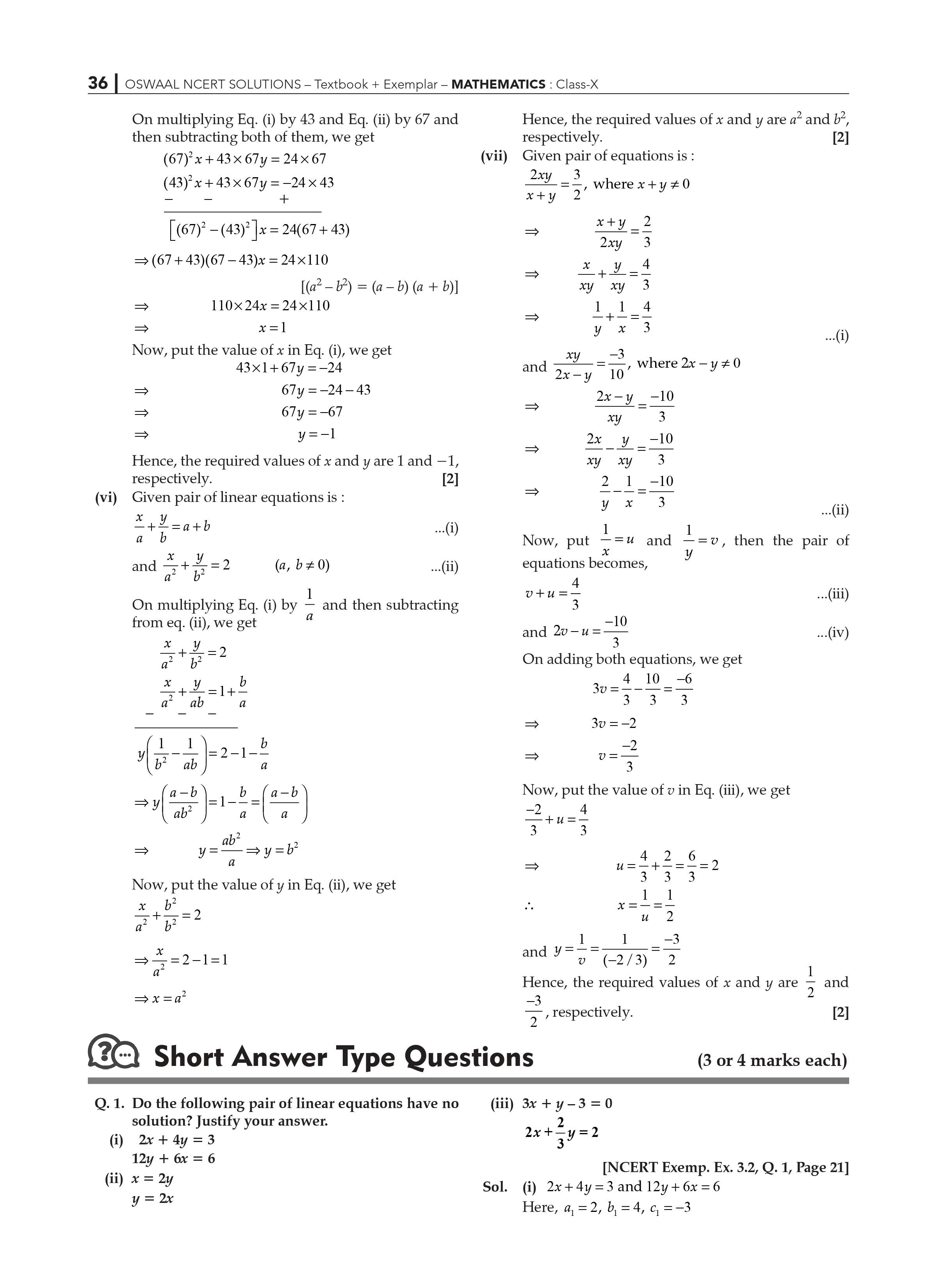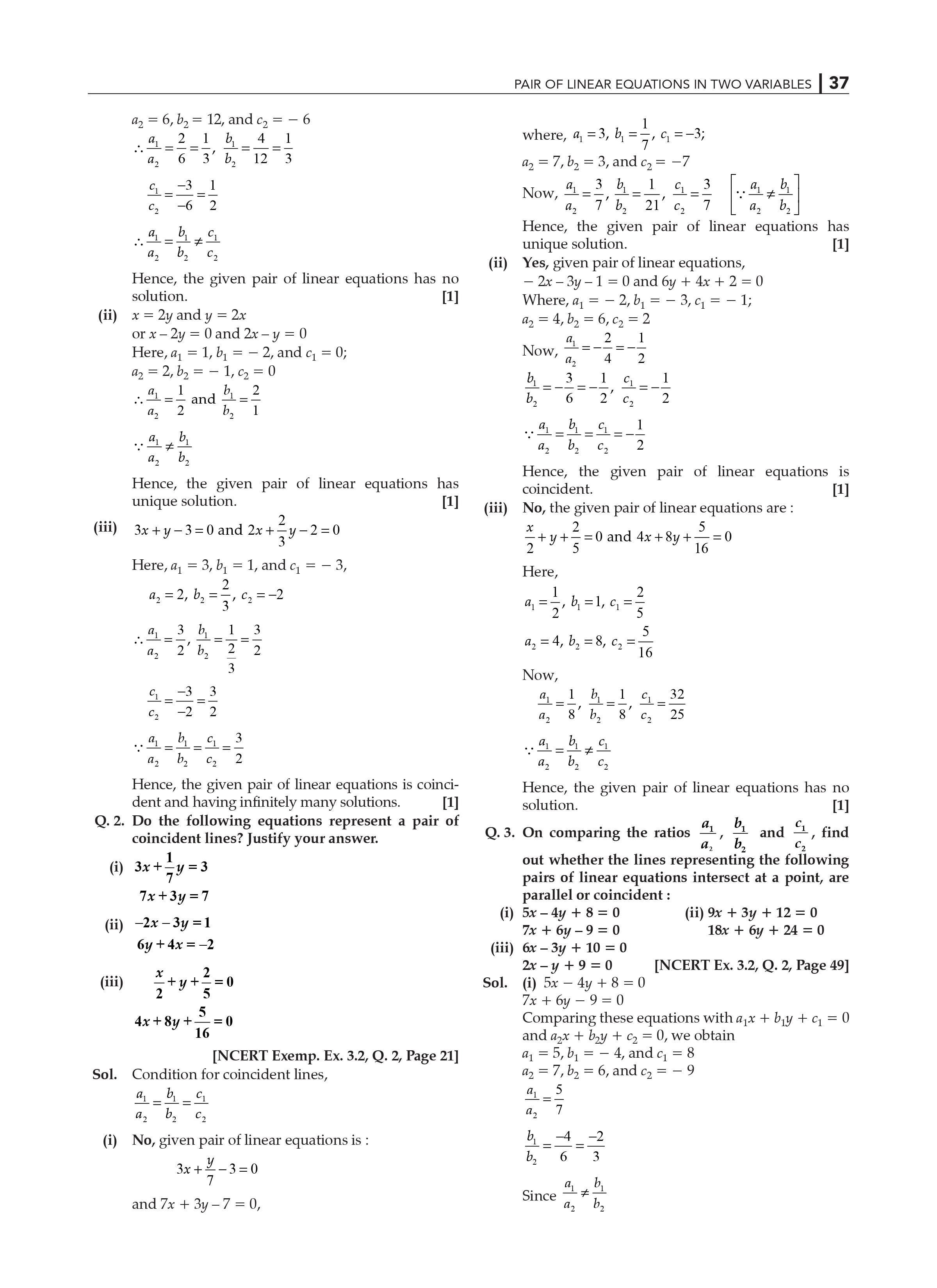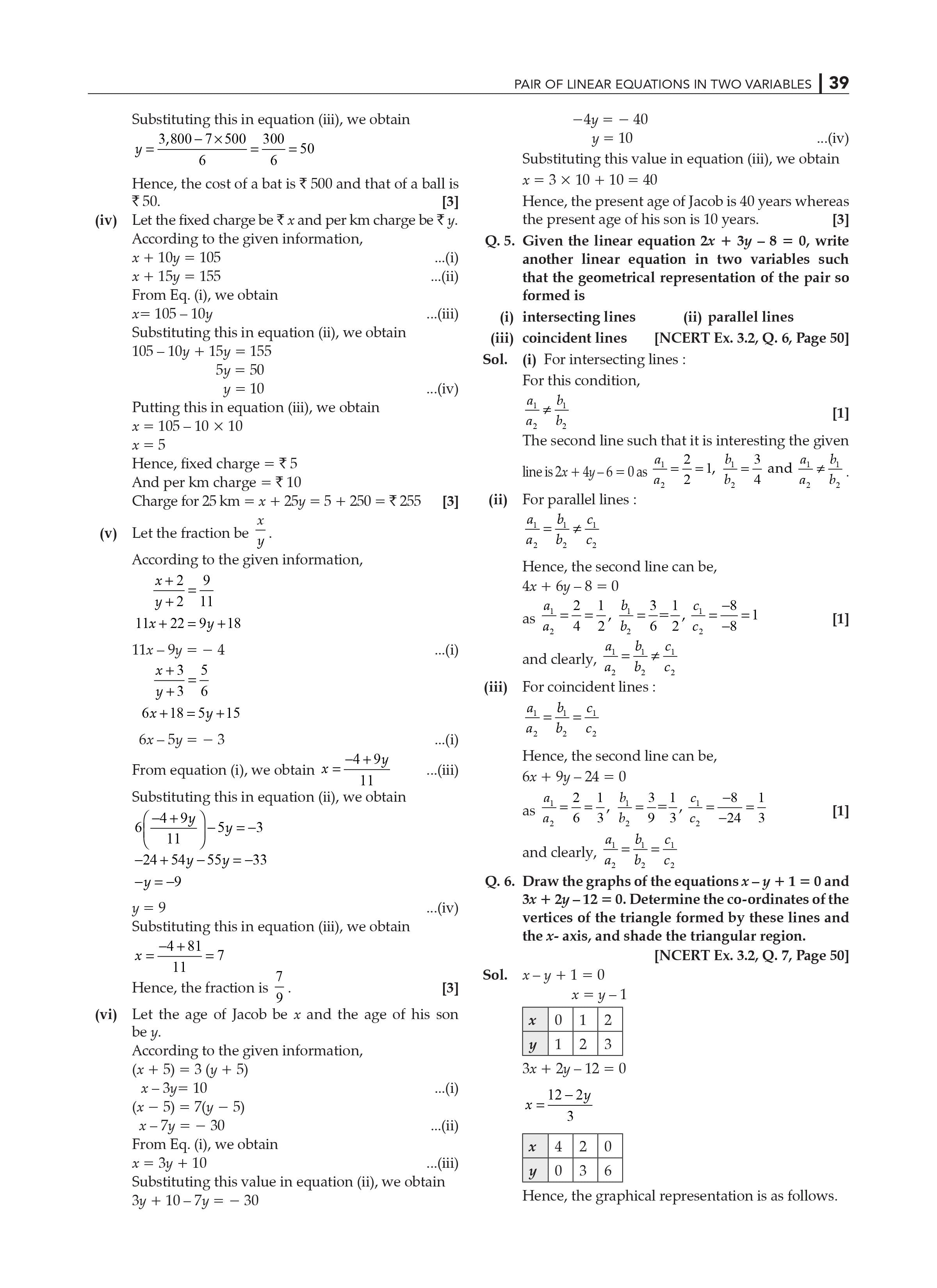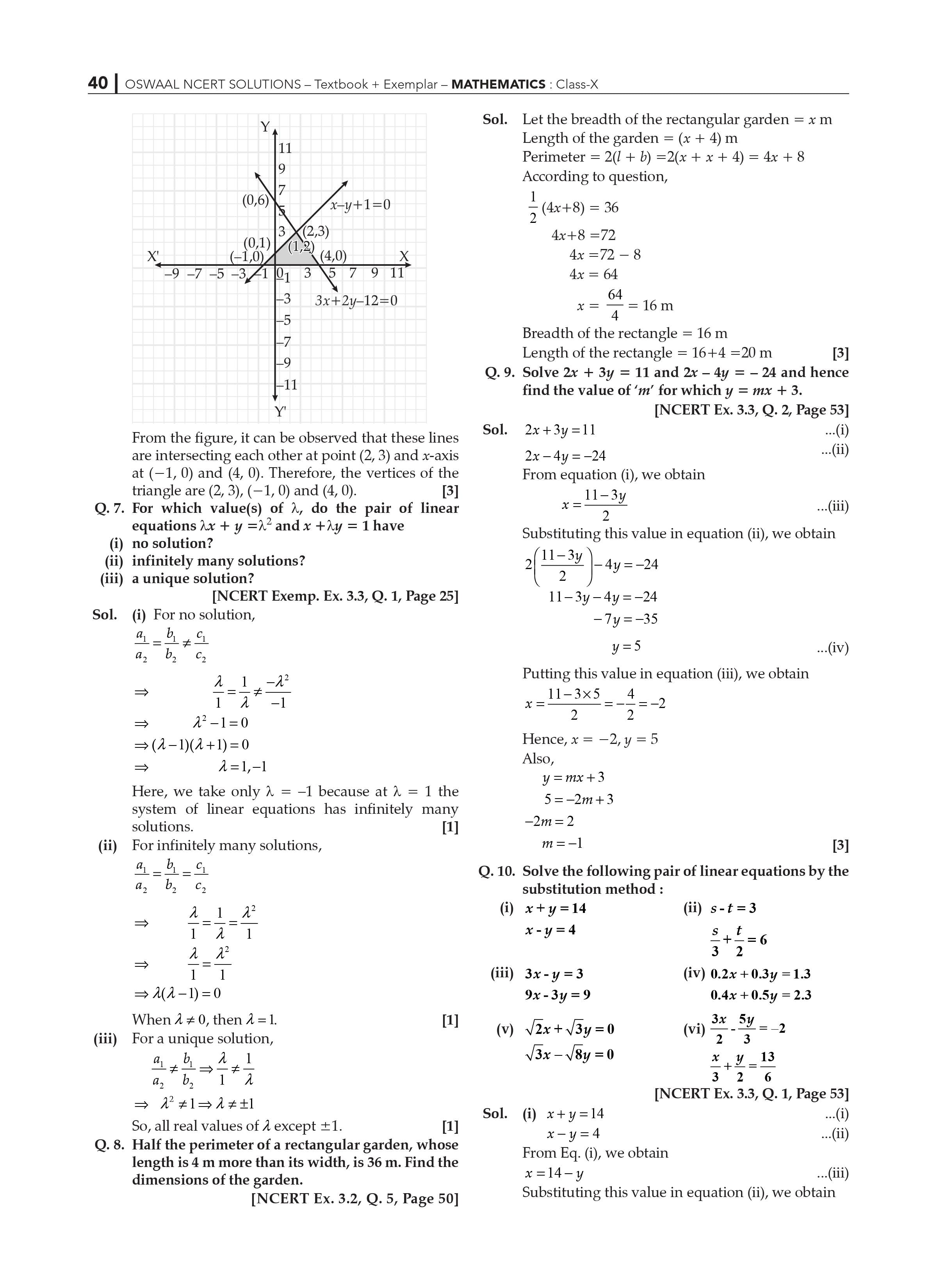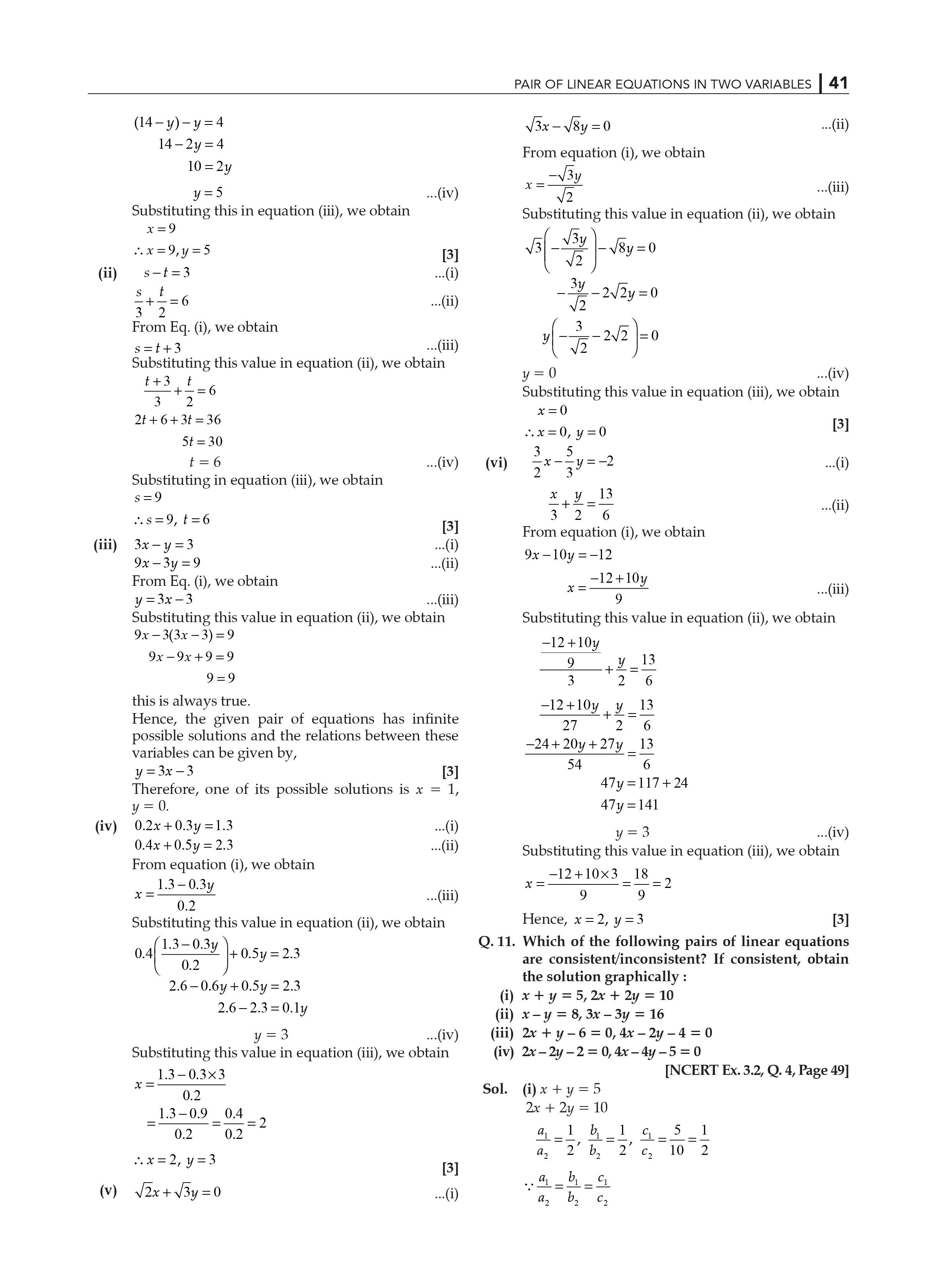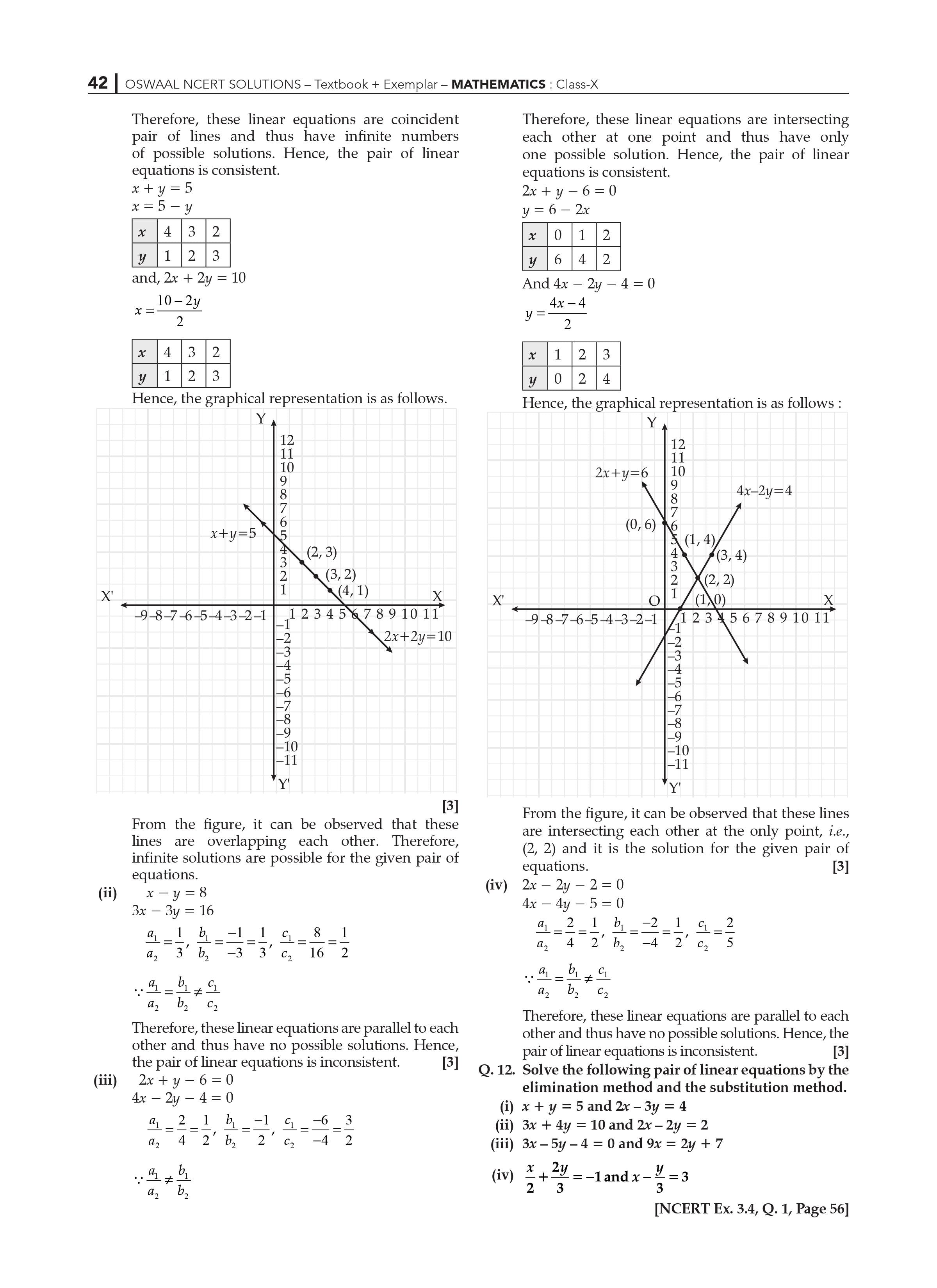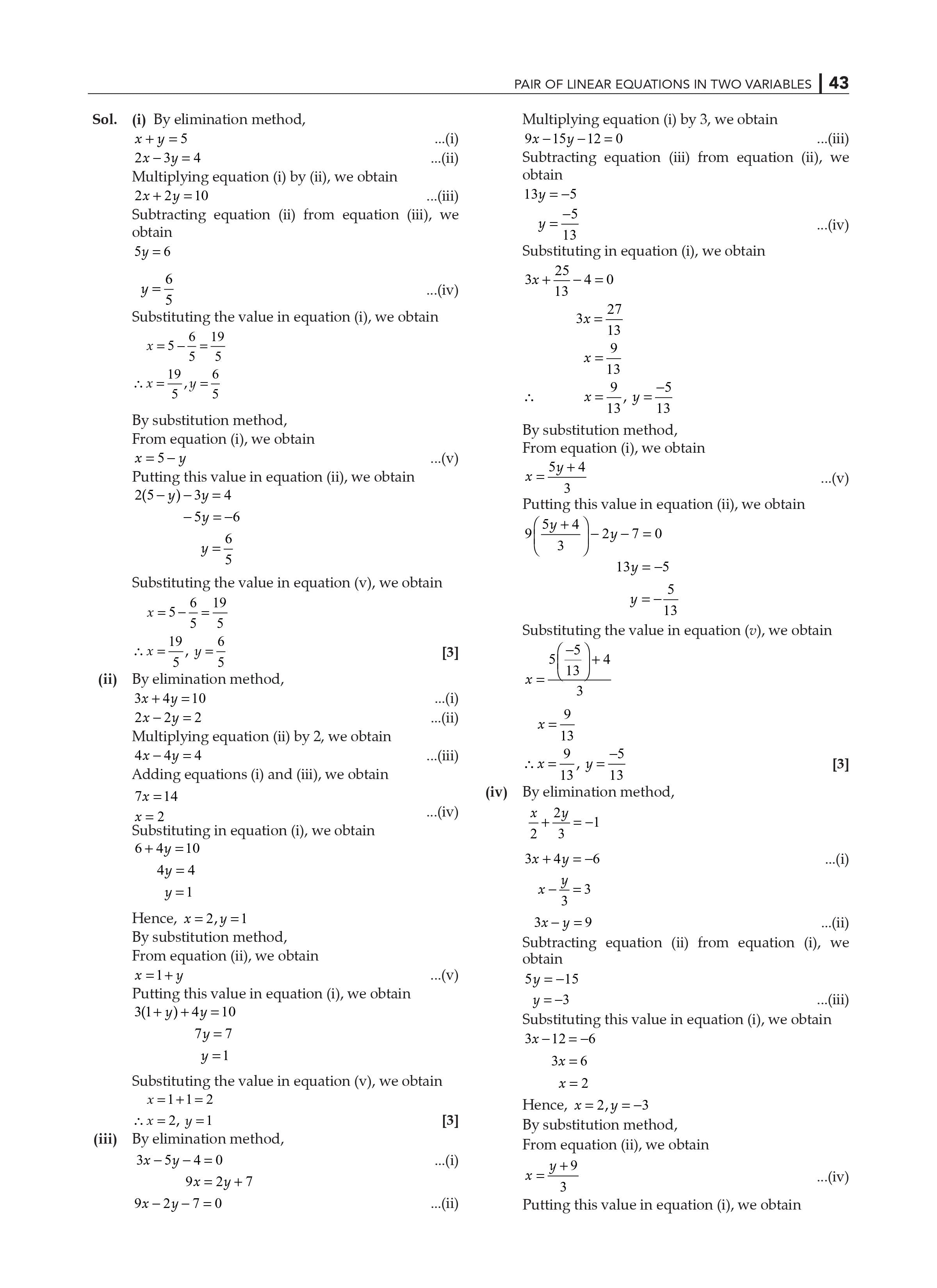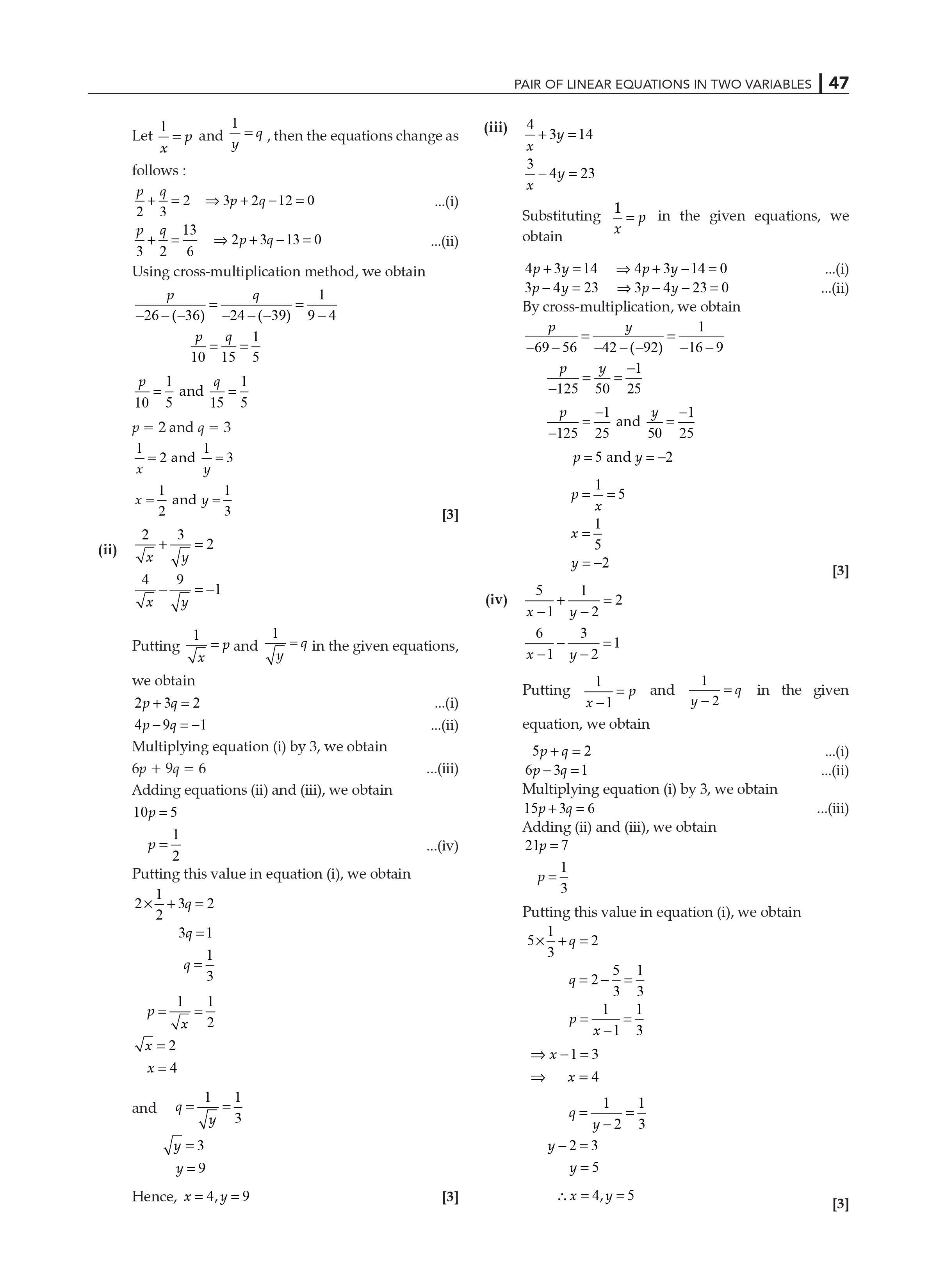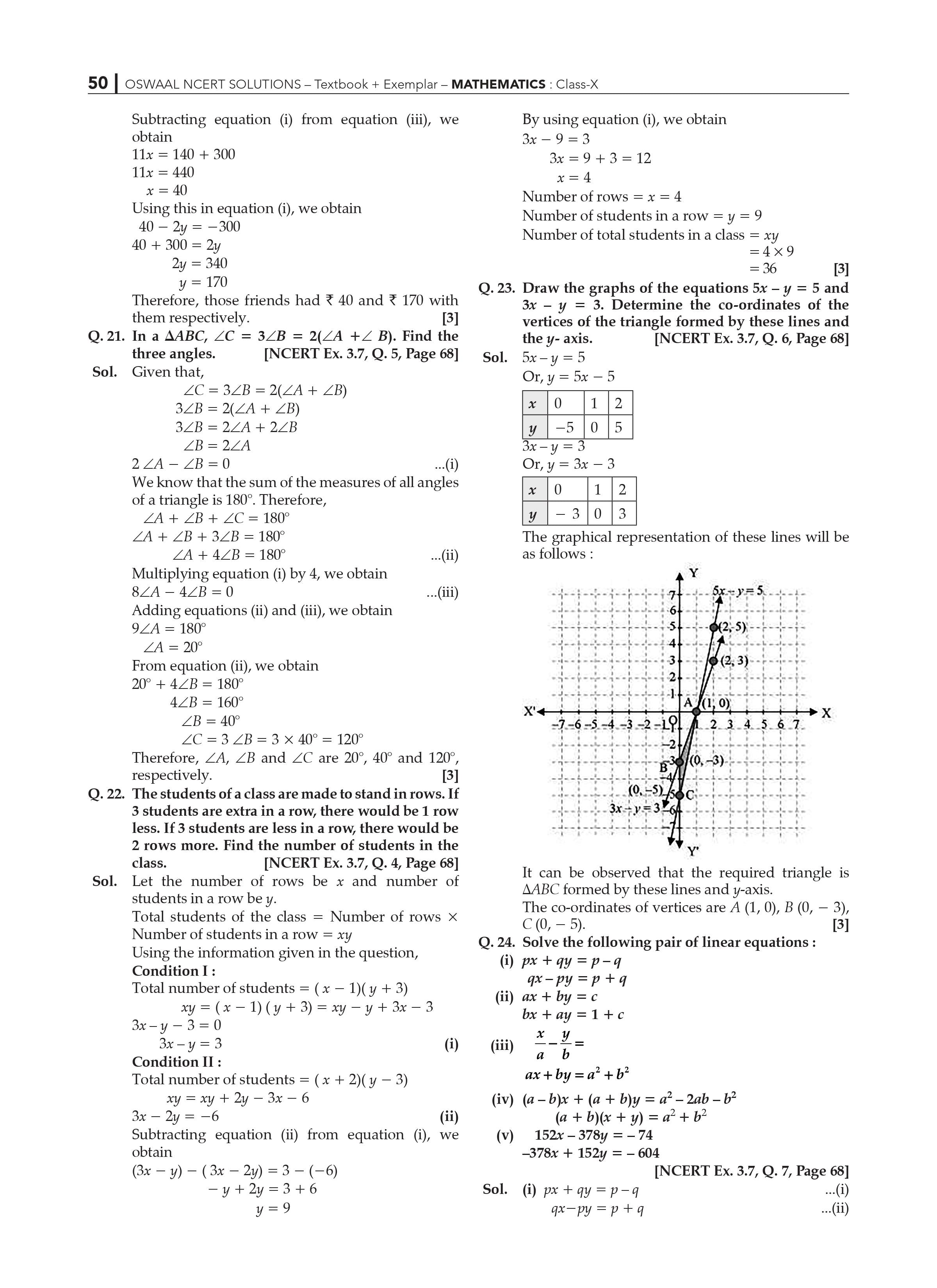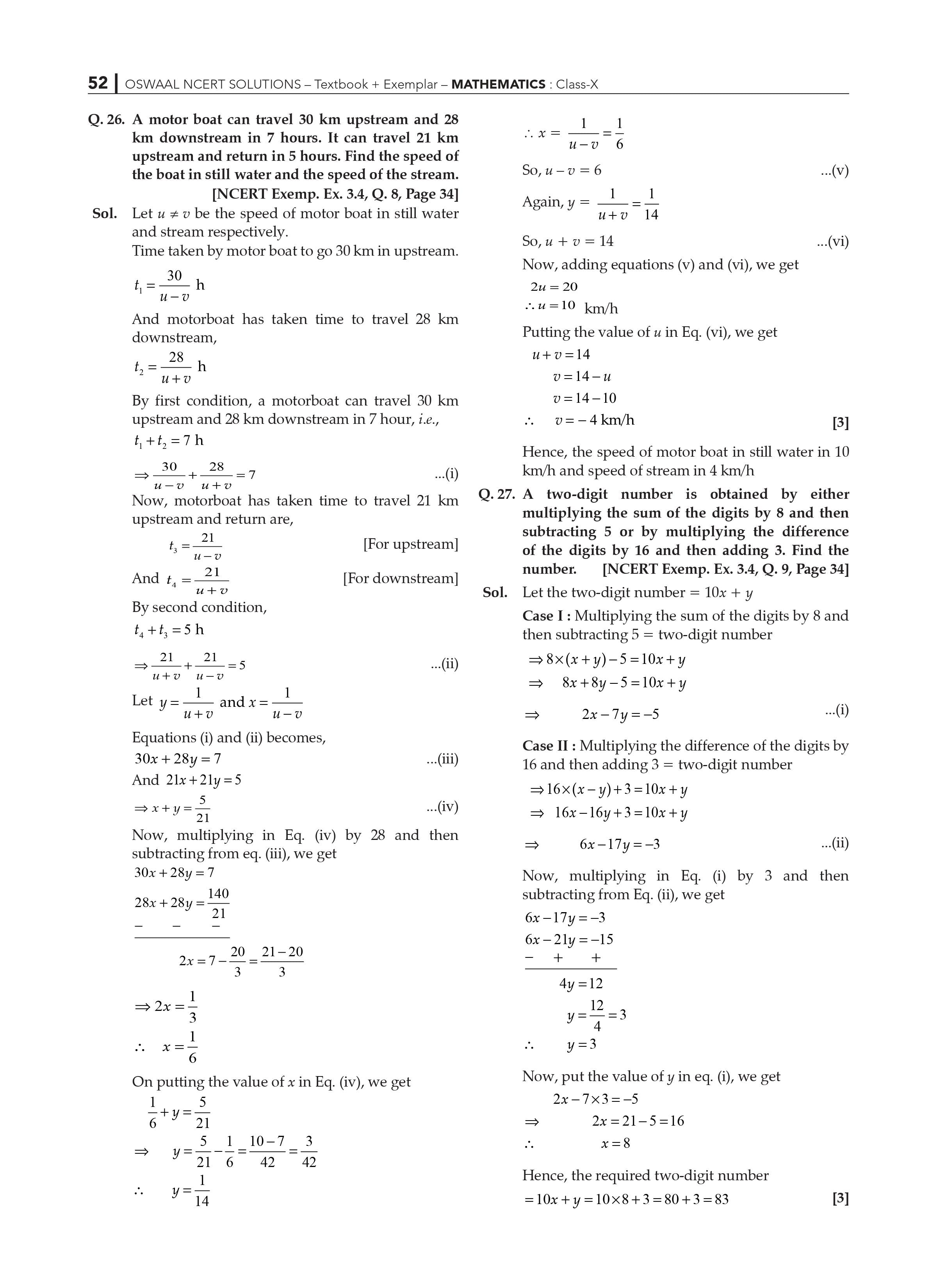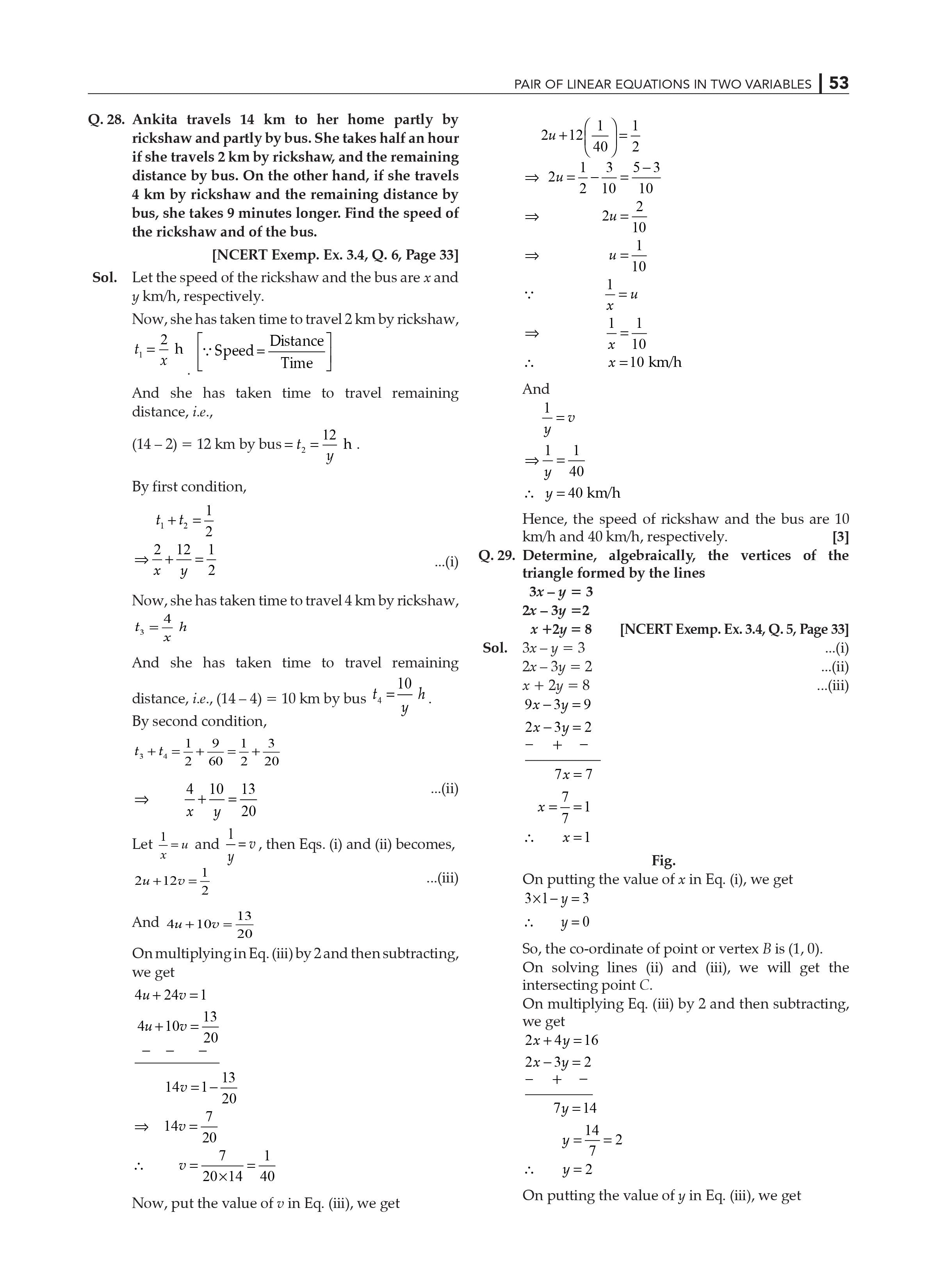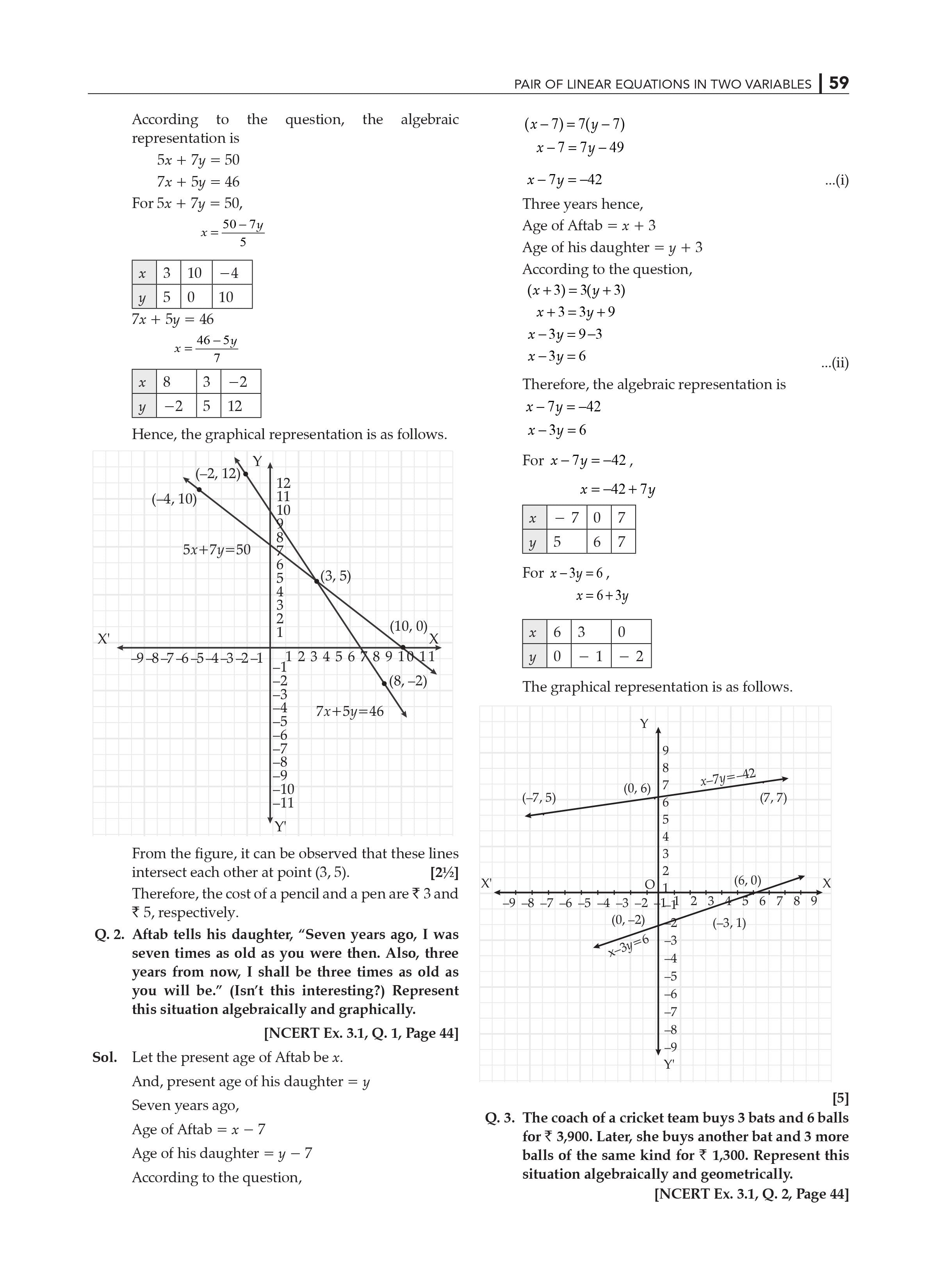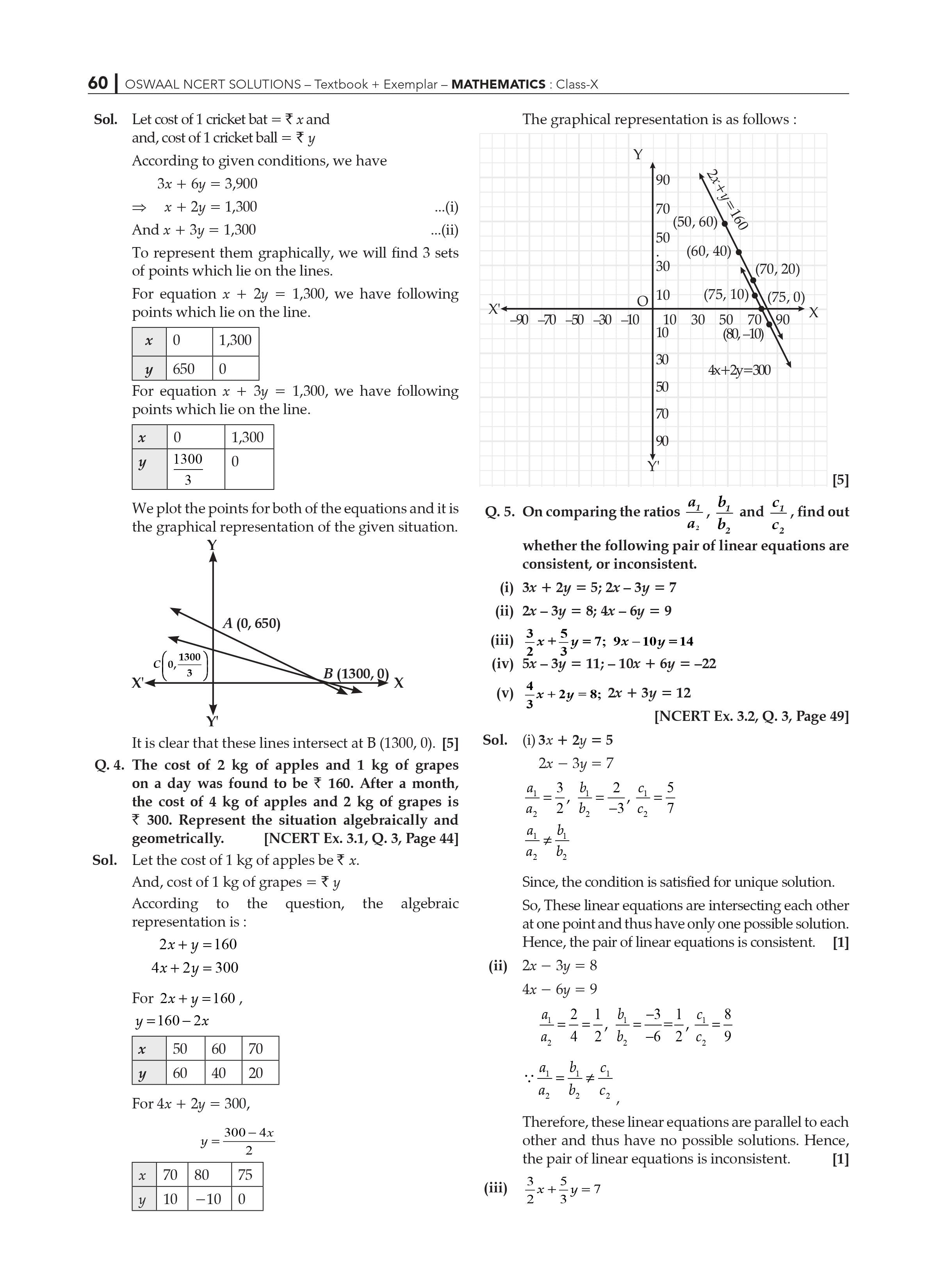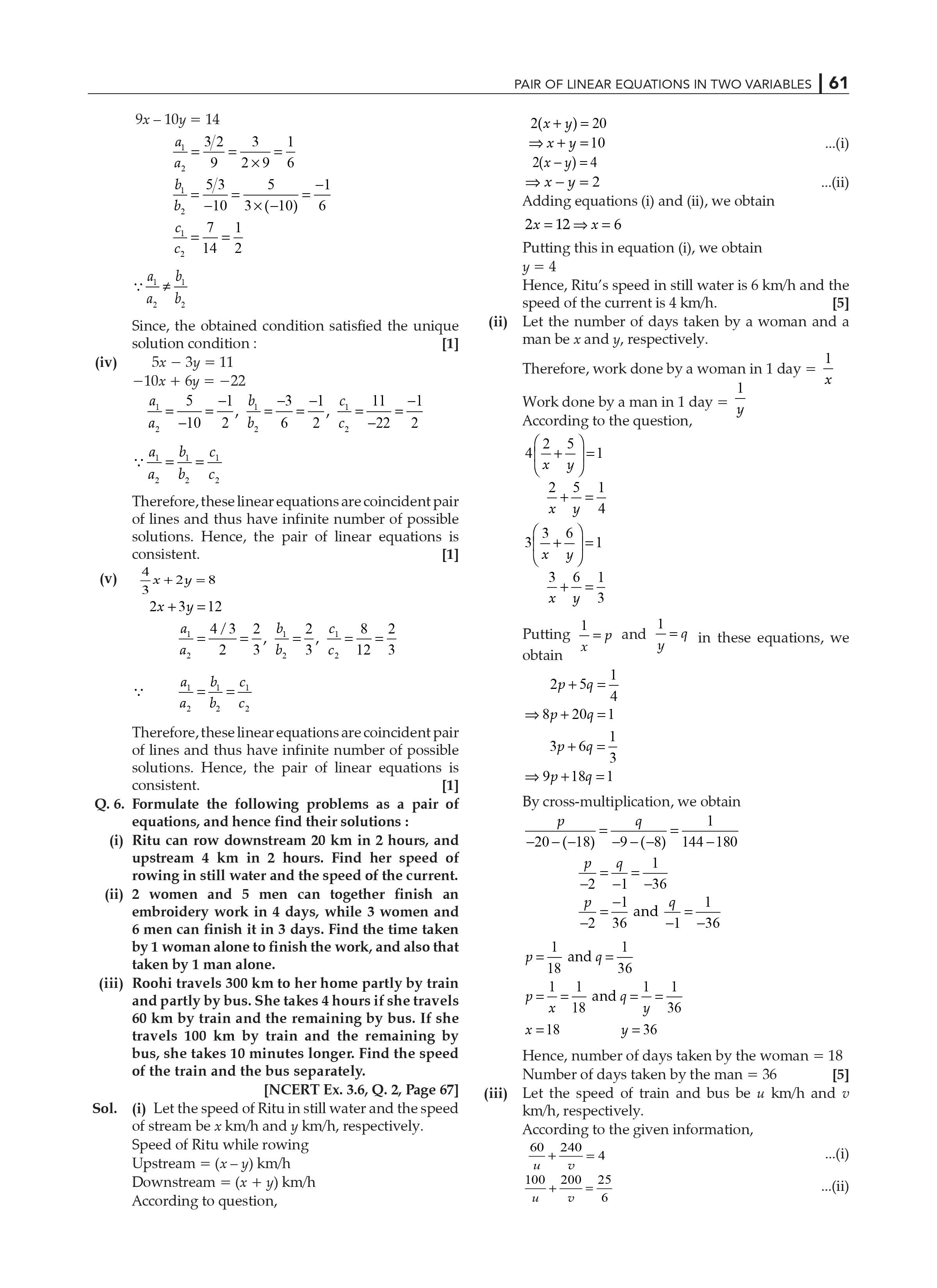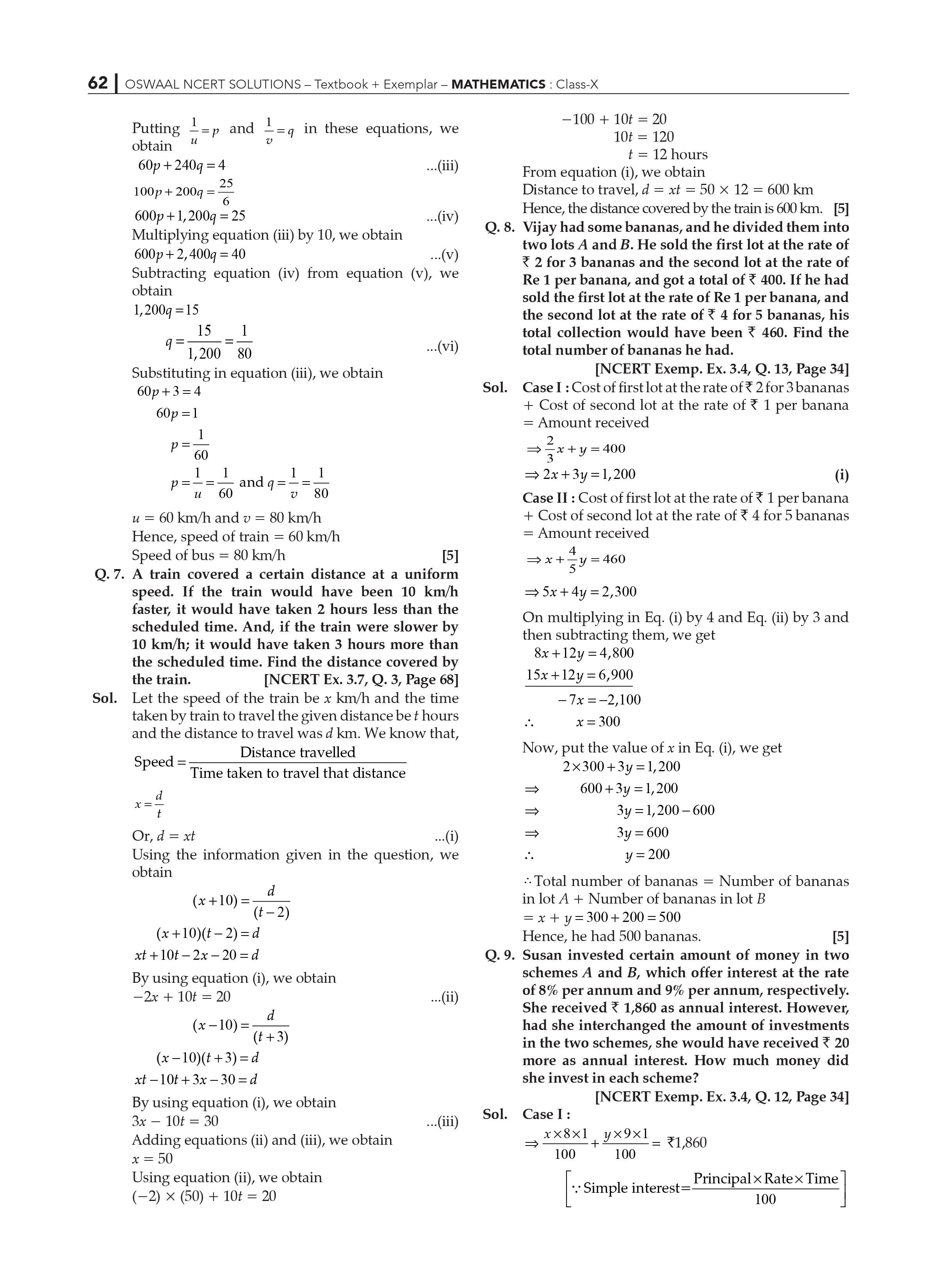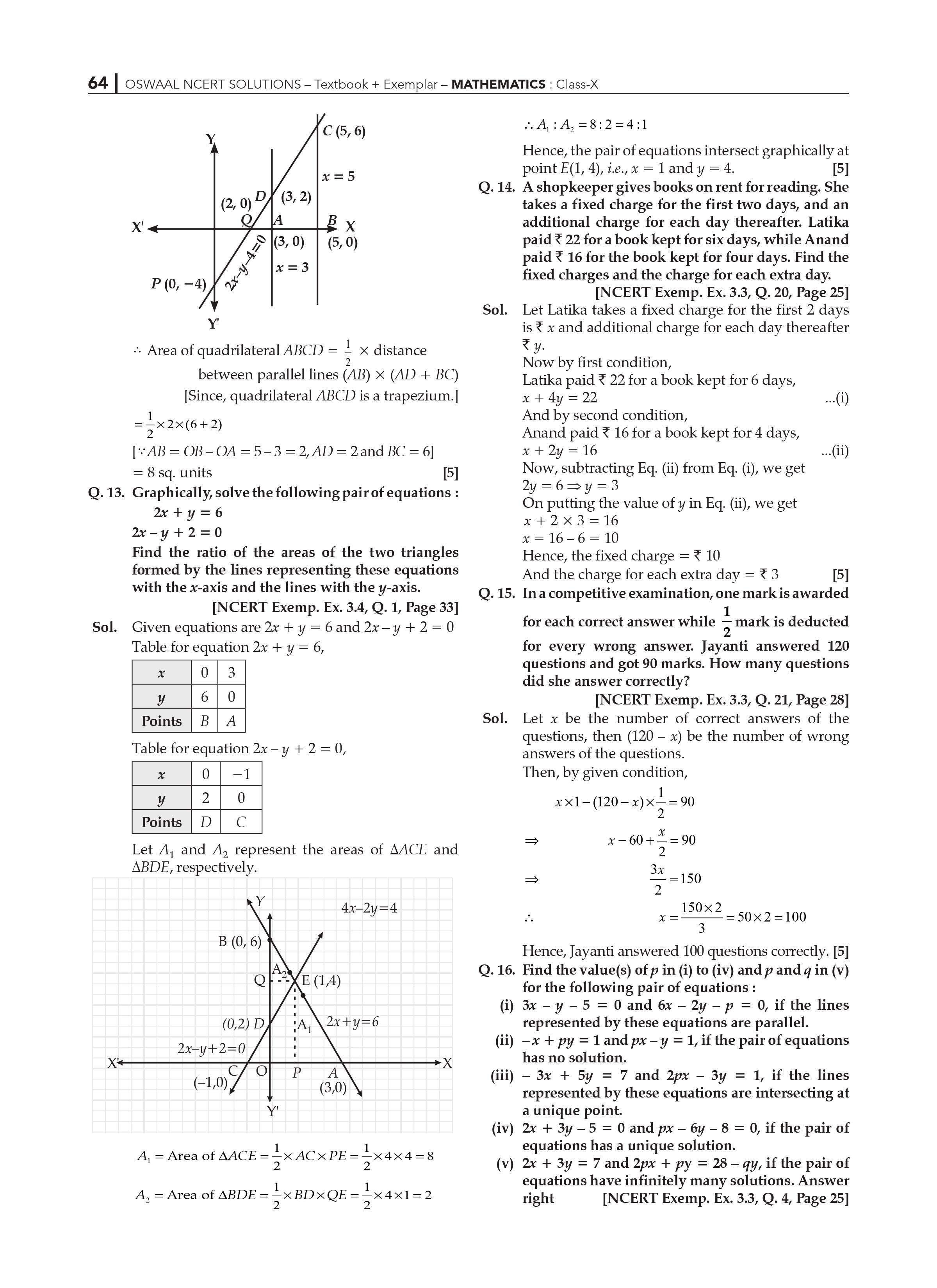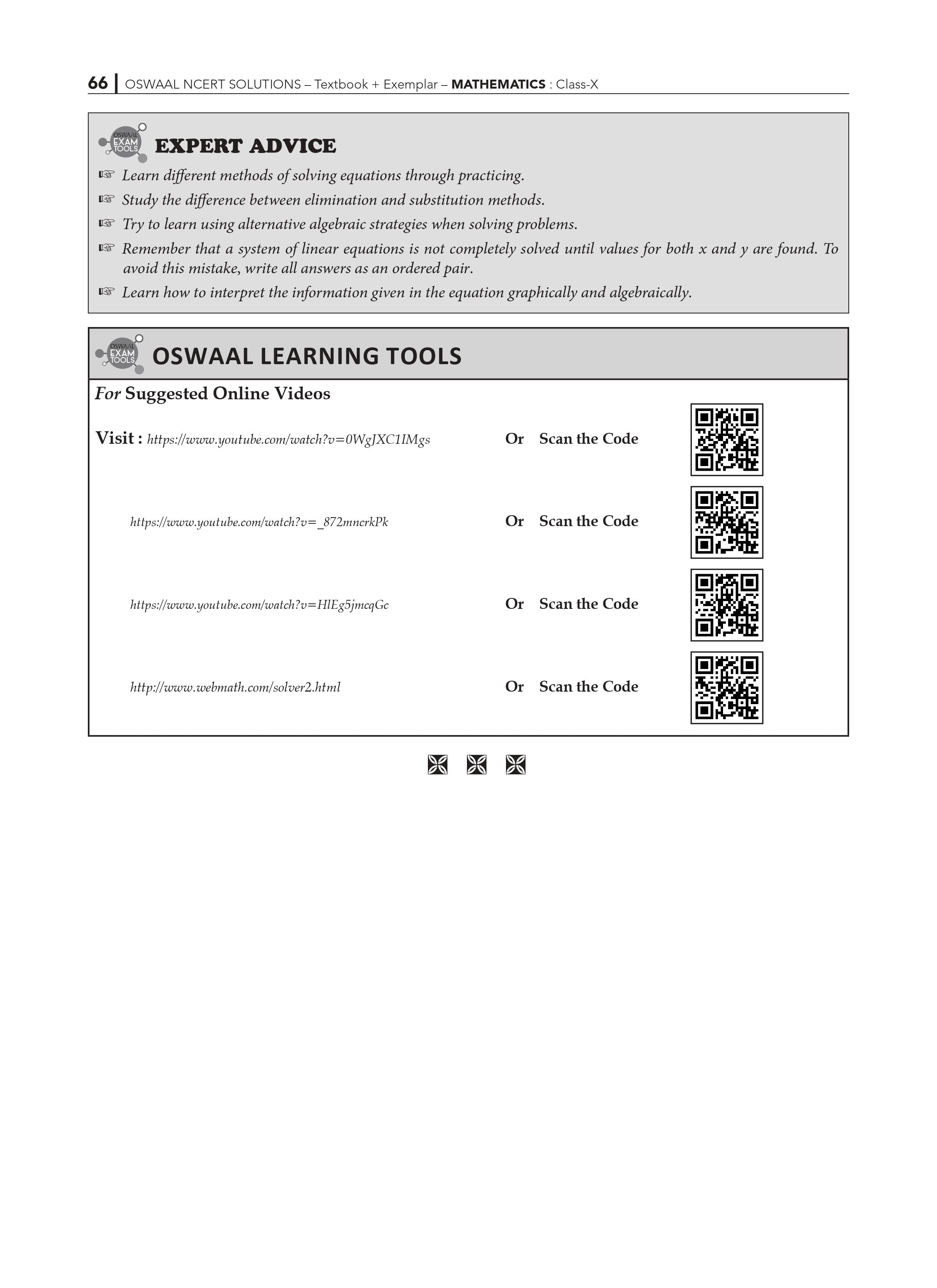NCERT Solutions for Class 10 Math Exercise 3.1 Chapter 3: Linear Equations in Two Variables

NCERT Solutions for Class 10 Math: The solutions to all of the questions of the textbook are included in Linear Equations in Two Variables. The topic of Pairs of Linear Variables is crucial for the exams. For better preparation, students are encouraged to use NCERT Solutions.

All of the Math problems are solved step by step in the NCERT Solutions Class 10 Math. These NCERT Solutions are the ideal resources for students studying with extensive study materials that cover all of the major themes. All of the relevant information has been included by the specialists. For a better grasp of the issue, students might refer to the solutions. It will be beneficial to students who are taking their tests.

This is the chapter's first exercise, and it consists of three questions. Subject experts provide solutions to this exercise in NCERT Solutions for Class 10 Maths Chapter 3 Pair of Linear Equations in Two Variables Exercise 3.1. Students can use this page to get step-by-step answers to the questions.

Students are requested to represent the supplied form of equations graphically or algebraically in all three questions.

Chapter 3: Pair of Linear Equations in Two Variables Exercise 3.1 is a key feature of NCERT Solutions for Class 10 Maths.

• NCERT Solutions for Class 10 Maths Chapter 3: Pair of Linear Equations in Two Variables NCERT Solutions for Class 10 Maths Chapter 3: Pair of Linear Equations in Two Variables Subject experts have framed Exercise 3.1.
• The answers given here are correct.
• The remedies.
• The answers given here are correct.

Students will benefit from the solutions as they prepare for their exams.

Chapter 3 Pair of Linear Equations in Two Variables Exercise 3.1

The first assignment in this chapter introduces the fundamentals of graphic and algebraic representation of linear equations. A straight line is the geometric depiction of a linear equation in two variables, as we know. The RD Sharma Solutions Class 10 teaches major ideas in this and other chapters that are important for students’ test preparation.

1. In terms of test preparation, what are the most important themes in NCERT Solutions for Class 10 Math Chapter 3, Exercise 3.1?

NCERT Solutions for Class 10 Math Chapter 3 covers the substitution method, elimination method, and cross-multiplication method for a pair of linear equations in two variables. Elimination and cross-multiplication are two methods for solving a pair of linear equations in two variables that can be used to solve problems. Completing problems based on each topic with examples and solutions might help students do well in their Class 10 2023 exams.

1. To solve pairs of linear equations in two variables, is it necessary to know all three procedures in NCERT Solutions for Class 10 Math Chapter 3, Exercise 3.1?

Yes, the NCERT Solutions for Class 10 Math Chapter 3 requires all three methods for solving a set of linear equations in two variables. This will be carried on in higher education, and they may appear in their Class 10 finals. Our experts explain these concepts in detail. As a result, the major Purpose of these experts-formulated solutions is to transmit knowledge on the fundamentals of math, which aids pupils in fully comprehending each concept.

1. How do I start reading NCERT Solutions for Class 10 Math Chapter 3?

Use this to get started.

To begin, learn and understand the definition of linear equations using the website. Then, on our website, review the responses provided by their experts. Students will obtain a thorough comprehension of all of the concepts taught in Chapter 3 of the NCERT Solutions for Class 10 Math by doing these tasks.

NCERT Solutions for Class 10 Maths Exercise 3.2 Chapter 3

NCERT Solutions for Class 10 Math Linear Equations in Two Variables is the most effective technique for pupils to correct their weaknesses. The answers are correct and come from topic specialists after a lot of brainstorming.

The NCERT Solutions for Class 10 Maths provide answers to all of the exercises in the textbook. It also includes a number of practice questions. These questions will aid students in their preparation for the 2023 exams. From the standpoint of the test, NCERT Solutions Chapter 3- Pair of Linear Equations in Two Variables is a crucial chapter. The students must be meticulous.

To solve the problems in this chapter, students must be well-versed in the fundamental ideas.

NCERT solutions serve as a reference during exams. They can be referred to by students if they have any questions. They can then practice and evaluate themselves, focusing on their weak regions.

This is the chapter's second exercise. There are seven questions in all. NCERT Solutions for Class 10 Maths contains the answers to these questions. Exercise 3.2 from Chapter 3: Pair of Linear Equations in Two Variables. Students can look up solutions on this page. The solutions are offered by topic specialists in a step-by-step manner and are error-free.

NCERT Solutions Class 10 Maths Chapter 3- Pair of Linear Equations in Two Variables: Key Features

• Experts in the field answer the questions.
• The responses are true and come after a lot of brainstorming.
• These may aid in achieving good results on the first-term exams.

NCERT Solutions for Class 10 Maths Chapter 3: Pair of Linear Equations in Two Variables include the following topics:

This exercise tests your understanding of how to generate graphs of linear equations while solving systems of simultaneous linear equations in two variables. Students can benefit much from the RD Sharma Solutions Class 10 in terms of eliminating their questions and preparing for exams.

1. What are the most essential themes in NCERT Solutions for Class 10 Math Chapter 3, Exercise 3.2 in terms of test preparation?

For a pair of linear equations in two variables, NCERT Solutions for Class 10 Math Chapter 3 covers the substitution approach, elimination method, and cross-multiplication method. Elimination and cross-multiplication are two problem-solving approaches for solving a pair of linear equations in two variables. Completing problems with examples and solutions based on each topic could help students do well on their Class 10 first and second semester exams.

Yes, all three approaches for solving a pair of linear equations in two variables are required by NCERT Solutions for Class 10 Math Chapter 3. This will continue in higher education, and they might even appear in their Class 10 finals. As a result, the primary goal of these Oswaal expert-formulated answers is to impart essential math knowledge to students, allowing them to grasp each subject completely.

1. How can I begin reading Chapter 3 of the NCERT Solutions for Class 10 Math?

To begin, use our website to discover and comprehend what linear equations are. Review the comments offered by their specialists on the website after that.

NCERT solution Chapter 3 Math class 10 Exercise 3.3 Linear equations in two variables.

All of the Maths problems are solved step by step in the NCERT Solutions Class 10 Maths. These are really useful for kids who are studying for board exams.

NCERT Solutions Class 10 Maths Chapter 3- Pair of Linear Variables is a critical topic for first-term exams and should be thoroughly covered. Students will do well in the first term exams if they practice the exercises frequently.

NCERT Solutions is the greatest study guide for students because it provides extensive study material that covers all of the major themes. The subject experts here provide accurate solutions. Experts have attempted

There are three questions in Exercise 3.3. NCERT Solutions for Class 10 Maths Chapter 3- Pair of Linear Equations in Two Variables contains the answers to these questions.

Exercising The answers can be found on this website for the pupils. In the NCERT Solutions, you’ll find solutions with detailed procedures. It would be beneficial to students in the event that they have any doubts.

Chapter 3: Pair of Linear Equations in Two Variables Exercise 3.3 is a key feature of NCERT Solutions for Class 10 Maths.?

The queries are answered by subject matter experts. As a result, the answers supplied are correct.

Here you will find the answers to all of the questions in the textbook.

The solutions will assist students in performing well on their first-term exams.

Chapter 3 Pair of Linear Equations in Two Variables Exercise 3.3 RD Sharma Solutions for Class 10 Maths?

We have algebraic methods in addition to graphical methods for solving simultaneous linear equations in two variables. There are three algebraic approaches for solving simultaneous linear equations in two variables. The main topics discussed in this exercise are method of elimination by substitution and method of elimination by equating the coefficients. For anything you can check

The NCERT Solutions Class 10 Maths Chapter 3- Pair of Linear Equations in Two Variables Exercise 3.4

The NCERT Solutions Class 10 Maths book provides step-by-step answers to all of the math questions in the textbook and elsewhere. To do well in the 2023 exams, students should practice the exercises several times.

NCERT Solutions is a comprehensive study guide that covers all of the major themes. Experts in the field have come up with precise solutions.

The NCERT Solutions Class 10 Maths Chapter 3- Pair of Linear Equations in Two Variables has a fourth exercise. It has two questions, the answers to which may be found in the NCERT Solutions Class 10 Maths book. If pupils have any questions, they can refer to these solutions.

Chapter 3: Pair of Linear Equations in Two Variables Exercise 3.4 is a key feature of NCERT Solutions for Class 10 Maths.?

The responses to the questions are correct.

You can easily find the answers to all of the questions in the textbook.

The queries are answered by subject matter experts.

Students can use NCERT Solutions for Class 10 Maths Chapter 3- Pair of Linear Equations in Two Variables Exercise 3.4 to help them do well in the first term exams.

Chapter 3 Pair of Linear Equations in Two Variables Exercise 3.4

In this group, the method of cross-multiplication is extensively examined. The RD Sharma Solutions Class 10 clearly explains how to solve systems of equations using the cross-multiplication approach. It's a wonderful resource created by our professionals to help students explain their worries and focus on their weaker areas.

NCERT Solutions for Class 10 Math Chapter 3 Pair of Linear Equations in Two Variables:

NCERT Solutions for Class 10 Math Exercise 3.5 Chapter 3

With Class 10 Maths NCERT Solutions, Students will be learning how to crack mathematical problems which Involve this concept in Chapter 3 Exercise 3.5 Pair of Linear Equations in Two Variables.

Mathematics is one of the most interesting subjects which demands a great amount of practice and focus. The students who are preparing to give the first and second term examinations in 10th class could utilize the NCERT Solutions Class 10 as a practice guide because NCERT Solutions enable the students to analyse themselves. Students can solve the exercises once they complete one chapter and interpret their weak points in the chapter. This helps them to work on and improve their shortcomings before they go to the examination hall.

The Exercise 3.5 of Chapter 3 Pair of Linear Equations in Two Variables solutions include proper stepwise solutions to all of the NCERT textbook's math questions.

A linear equation involving two variables x & y is an equation of the pattern ax + by + c = 0, where a, b, and c are real values and a and b are not both zero. a & b are called the coefficient of x and y respectively.

The NCERT Solutions for Class 10 Mathematics Chapter 3 moreover illustrates that a solution to an equation like this is a set of values, one for x and the other for y, that balance the two sides, such as L.H.S. and R.H.S, of the equation. Students further learn that each equation answer is represented by a point on a line.

NCERT Solutions for Class 10 Math Chapter 3 – Pair of Linear Equations in Two Variables NCERT Solutions for Class 10 Math Chapter 3 – Pair of Linear Equations in Two Variables.

In the tests, Chapter 3- Pair of Linear Equations in Two Variables is worth 11 points. This chapter covers a wide range of issues concerning linear equations in two variables.

NCERT solutions for chapter 3 exercise 3.5 in class 10 maths. The cross-multiplication method of solving linear equations is introduced in Pair of Linear Equations in Two Variables. This method is thoroughly explained using the real-world example of oranges and apples, where the cost is calculated using this concept. NCERT solutions class 10 maths chapter 3 exercise 3.5 is made up of two questions with numerous subparts.

If a pair of linear equations a1 x + b1 y + c 1 = 0 and a2 x + b2 y + c 2 = 0, the general form of the x and y variables using the cross-multiplication method is as follows:

x/(b1c2 - b2c1) = y/(c1a2 - c2a1) = 1 (a1b2 - a2b1)

As a result, the following steps can be used to solve the cross-multiplication problem.

• Put the given equations in standard form.
• Use the notation shown above, or cross multiply the values.
• Find x and y if (a1b2 - a2b1) = 0.

NCERT solutions for class 10 mathematics chapter three, exercise 3.5 A set of solved examples of equations reducible to a pair of linear equations in two variables is referred to as a pair of linear equations in two variables. Students must make an effort to practise them on their own in order to identify and correct their errors.

The cross-multiplication method is discussed in NCERT solutions for class 10 maths chapter 3 exercise 3.5, which kids must practise using a cross-multiplication diagram while multiplying both equations. The cross-multiplication diagram can be a confusing step, so it is critical that children practise it on a regular basis so that they do not make silly mistakes during an examination. NCERT solutions for class ten maths chapter three, exercise 3.5 A set of linear equations in two variables is included in pairs of linear equations.

NCERT Solutions Class 10 Maths Chapter 3- Pair of Linear Equations in Two Variables Exercise 3.5 Key Features

• The responses to the questions are correct.
• All of the answers to the textbook's questions can be found here.
• The questions are answered by subject matter experts.
• NCERT Solutions for Class 10 Maths Chapter 3- Pair of Linear Equations in Two Variables Exercise 3.5 can assist students in performing well in their first term exams.

1. In terms of exam preparation, what are the most important themes in NCERT Solutions for Class 10 Math Chapter 3?

1. NCERT Solutions for Class 10 Math Chapter 3 covers the substitution method, elimination method, and cross-multiplication method of a pair of linear equations in two variables. Methods for solving a pair of linear equations in two variables include elimination and cross-multiplication. Students in Class 10 can do well in their first and second term exams by completing problems based on each topic with examples and solutions.

1. Is it necessary to comprehend all three strategies in NCERT Solutions for Class 10 Math Chapter 3 to solve pairs of two-variable linear equations?

1. Yes, in NCERT Solutions for Class 10 Math Chapter 3, all three methods for solving a set of linear equations in two variables are required. This will be continued in higher education, and they may appear in their Class 10 finals. We describe these ideas in great detail. As a result, the primary goal of these expert-formulated answers is to impart knowledge on the fundamental parts of math, allowing students to properly understand each concept.

1. How do I get started with Chapter 3 of NCERT Solutions for Class 10 Math?
2. To begin, use the website to learn and practice.

understand the meaning of linear equations Then, on our website, review the responses provided by their professionals. Students will gain a thorough understanding of all of the concepts covered in Chapter 3 of the NCERT Solutions for Class 10 Math by completing these activities.

NCERT Solutions for Class 10 Math Exercise 3.6 of Chapter 3.

NCERT Solutions for Class 10 Mathematics in Chapter 3 Exercise 3.6 Pairs of Linear Equations in Two Variables, students will learn how to solve mathematical problems that involve this concept.

Mathematics is a fascinating subject that necessitates a great deal of practice and concentration. Students preparing to take Class 10 could use the NCERT Solutions Class 10 as a practice guide because NCERT Solutions allow students to analyse themselves. After completing one chapter, students can solve the exercises and interpret their weak points in the chapter. This makes it easier for them to work on and improve their shortcomings before they go to the examination hall.

The solutions to Exercise 3.6 of Chapter 3 Pair of Linear Equations in Two Variables include correct step-wise solutions to all of the NCERT textbook's math questions.

A linear equation with two variables x and y is an equation of the form ax + by + c = 0, where a, b, and c are all real numbers and a and b are not both zero. a and b are known as the coefficients of x and y, respectively.

The NCERT Solutions for Class 10 Mathematics Chapter 3 also shows that a solution to an equation like this is a set of values, one for x and the other for y, that balance the two sides of the equation, such as L.H.S. and R.H.S. Students are also taught that each equation answer is represented by a point on a line.

NCERT Solutions for Class 10 Maths Exercise 3.6 Linear Equations in Two Variables contains in-depth study material for the topics covered in the textbook. NCERT Solutions Class 10 Maths Chapter 3- Pair of Linear Variables is mentioned in the NCERT Solutions, along with the answers to the textbook exercises. NCERT Solutions Class 10 Maths Chapter 3- Pair of Linear Equations in Two Variables Exercise 3.6 contains solutions to the chapter's problems.

NCERT Solutions Class 10 Maths provides step-by-step solutions to all of the Math problems asked in the textbook. These exercises should be practised by the students in order to identify their flaws.

The solutions provided by subject experts are contained in NCERT Solutions. These serve as a guide for students preparing for their first term exams They can be referred to by students if they have any questions.

NCERT Solutions for Chapter 3 Exercise 3.6 in Class 10 Maths Pairs of Linear Equations in Two Variables teaches students about another concept related to linear equations in two variables: equations reducible to a pair of linear equations in two variables. Because this is a new topic that can be a little tricky, students must go over the theory twice. This section deals with nonlinear equations that are reduced to a linear form by using appropriate substitutions. NCERT Solutions Class 10 maths chapter 3 exercise 3.6 is an interesting exercise with questions based on real-life examples.

In this exercise, the majority of the variables are in fractional form, indicating that they are nonlinear. This exercise consists of two questions, each with a subpart. The first is quite simple because it requires direct substitutions of the mathematical expression, whereas the second involves word problems. This is the difficult part of the exercise that puts a child's knowledge to the test.

There are mainly 2 types of questions that are asked from this exercise in the examination hall.

Tips to do better in this exercise:

NCERT Solutions Class 10 Maths Chapter 3 Exercise 3.6 Pairs of Linear Equations in Two Variables teaches students how to replace fractional terms with a different variable. In this manner, an equation becomes linear and can be solved in a standard manner. When they have the solution, they can reintroduce the fraction and obtain the variable values.

NCERT Solutions Class 10 Maths Chapter 3- Pair of Linear Equations in Two Variables Exercise 3.6 Key Features

• The responses to the questions are correct.
• All of the answers to the textbook's questions can be found here.
• The questions are answered by subject matter experts.
• NCERT Solutions for Class 10 Maths Chapter 3- Pair of Linear Equations in Two Variables Exercise 3.6 can assist students in performing well in their first term exams.

1. In terms of exam preparation, what are the most important themes in NCERT Solutions for Class 10 Math Chapter 3?
2. NCERT Solutions for Class 10 Math Chapter 3 covers the substitution method, elimination method, and cross-multiplication method of a pair of linear equations in two variables. Methods for solving a pair of linear equations in two variables include elimination and cross-multiplication. Students in Class 10 can do well in their first and second term exams by completing problems based on each topic with examples and solutions.

1. Is it necessary to comprehend all three strategies in NCERT Solutions for Class 10 Math Chapter 3 to solve pairs of two-variable linear equations?

Yes, in NCERT Solutions for Class 10 Math Chapter 3, all three methods for solving a set of linear equations in two variables are required. This will be continued in higher education, and they may appear in their Class 10 finals. We describe these ideas in great detail. As a result, the primary goal of these expert-formulated answers is to impart knowledge on the fundamental parts of math, allowing students to properly understand each concept.

1. How do I get started with Chapter 3 of NCERT Solutions for Class 10 Math?

To begin, use our website to learn and practice.

understand the meaning of linear equations Then, on the website, review the responses provided by their professionals. Students will gain a thorough understanding of all of the concepts covered in Chapter 3 of the NCERT Solutions for Class 10 Math by completing these activities.